# Finding distances between points that share a common coordinate given the graph Online Quiz

Following quiz provides Multiple Choice Questions (MCQs) related to Finding distances between points that share a common coordinate given the graph. You will have to read all the given answers and click over the correct answer. If you are not sure about the answer then you can check the answer using Show Answer button. You can use Next Quiz button to check new set of questions in the quiz.Q 1 - Find the distance between the pair of points in the following graph: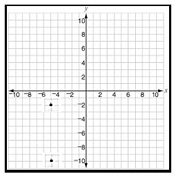### Explanation

Step 1 − The coordinates of the pair of points are (−5, −2) and (−5, −10).

Step 2 − The distance between the pair of points is the difference between their y coordinates as x coordinates are same.

Step 3 − The distance between the pair of points = −2 –(−10) = −2 + 10 = 8 units.

Q 2 - Find the distance between the pair of points in the following graph: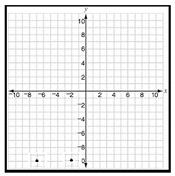### Explanation

Step 1 − The coordinates of the pair of points are (−2, −10) and (−7, −10).

Step 2 − The distance between the pair of points is the difference between their x coordinates as y coordinates are same.

Step 3 − The distance between the pair of points = −2 –(−7) = −2 + 7 = 5 units.

Q 3 - Find the distance between the pair of points in the following graph: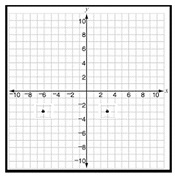### Explanation

Step 1 − The coordinates of the pair of points are (3, −3) and (−6, −3).

Step 2 − The distance between the pair of points is the difference between their x coordinates as y coordinates are same.

Step 3 − The distance between the pair of points = 3 –(−6) = 3 + 6 = 9 units.

Q 4 - Find the distance between the pair of points in the following graph:### Explanation

Step 1 − The coordinates of the pair of points are (5, 4) and (−4, 4).

Step 2 − The distance between the pair of points is the difference between their x coordinates as y coordinates are same.

Step 3 − The distance between the pair of points = 5 –(−4) = 5 + 4 = 9 units.

Q 5 - Find the distance between the pair of points in the following graph: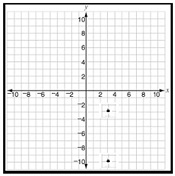### Explanation

Step 1 − The coordinates of the pair of points are (3, −3) and (3, −10).

Step 2 − The distance between the pair of points is the difference between their y coordinates as x coordinates are same.

Step 3 − The distance between the pair of points = −3 –(−10) = −3 + 10 = 7 units.

Q 6 - Find the distance between the pair of points in the following graph: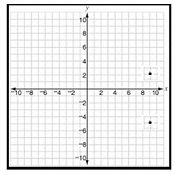### Explanation

Step 1 − The coordinates of the pair of points are (9, 2) and (9, −5).

Step 2 − The distance between the pair of points is the difference between their y coordinates as x coordinates are same.

Step 3 − The distance between the pair of points = 2 –(−5) = 2 + 5 = 7 units

Q 7 - Find the distance between the pair of points in the following graph: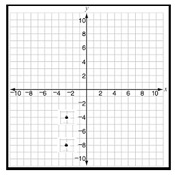### Explanation

Step 1 − The coordinates of the pair of points are (−3, −4) and (−3, −8).

Step 2 − The distance between the pair of points is the difference between their y coordinates as x coordinates are same.

Step 3 − The distance between the pair of points = −4 –(−8) = −4 + 8 = 4 units.

Q 8 - Find the distance between the pair of points in the following graph: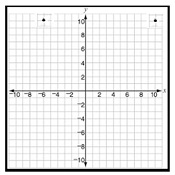### Explanation

Step 1 − The coordinates of the pair of points are (−6, 10) and (10, 10).

Step 2 − The distance between the pair of points is the difference between their x coordinates as y coordinates are same.

Step 3 − The distance between the pair of points = 10 – (−6) = 10 + 6 = 16 units

Q 9 - Find the distance between the pair of points in the following graph: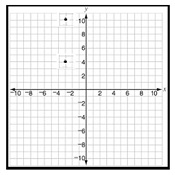### Explanation

Step 1 − The coordinates of the pair of points are (−3, 10) and (−3, 4).

Step 2 − The distance between the pair of points is the difference between their y coordinates as x coordinates are same.

Step 3 − The distance between the pair of points = 10 – 4 = 6 units.

Q 10 - Find the distance between the pair of points in the following graph: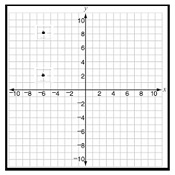### Explanation

Step 1 − The coordinates of the pair of points are (−6, 2) and (−6, 8).

Step 2 − The distance between the pair of points is the difference between their y coordinates as x coordinates are same.

step 3 − The distance between the pair of points = 8 – 2 = 6 units

finding_distances_between_points_that_share_common_coordinate_given_graph.htm